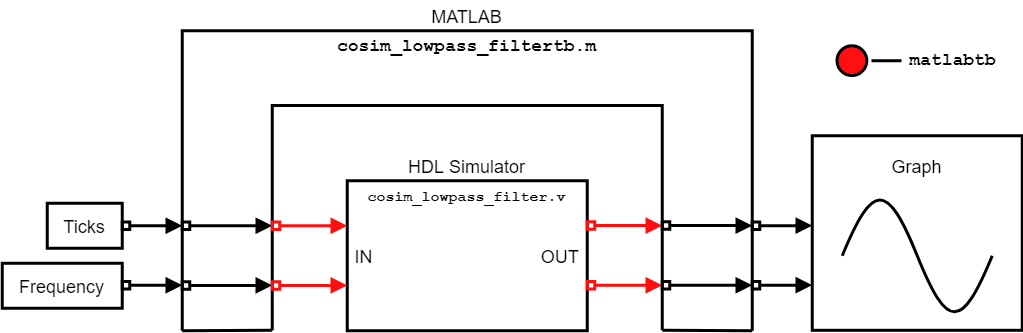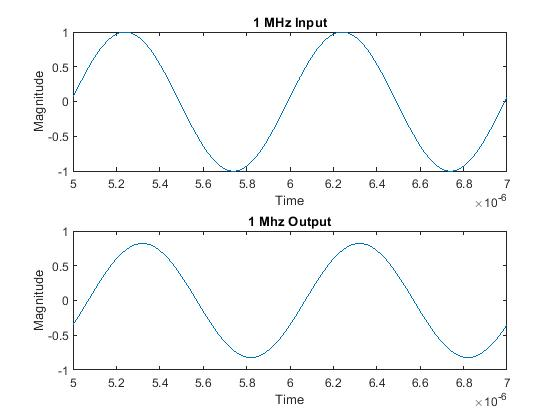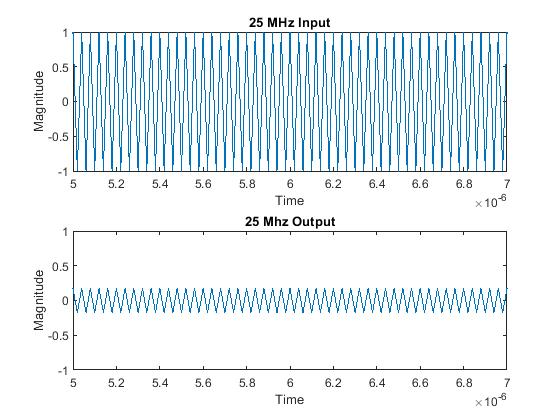# Cosimulation for Testing Filter Component Using MATLAB Test Bench

This example shows how to run either Mentor Graphics® ModelSim®/Questasim® or Cadence® Xcelium® with MATLAB® in batch mode to test an HDL component using a MATLAB test bench, by using the HDL Verifier™ function `matlabtb`. The function `matlabtb` facilitates this testing by feeding MATLAB-generated input data into the HDL component and feeding the output from the component back into MATLAB. When you generate complex waveforms in HDL, using `matlabtb` enables you to spend more time running tests than writing test waveforms. `matlabtb` also reads the results, so the testing process can leverage MATLAB to apply data transformations to the output before plotting. For more information on writing test benches for cosimulation, see Create a MATLAB Test Bench.

In this example, MATLAB generates a sine wave with an adjustable frequency, which is fed into a low-pass filter implemented in Verilog®. `matlabtb` passes the filter outputs to MATLAB for plotting. This process of testing is possible with MATLAB and with the HDL simulator in command-line mode. This diagram shows an overview of the simulation.This example considers the point of view of an HDL developer, so the main emphasis of the example is on using the HDL simulator and command-line terminal to perform testing and using MATLAB as an aid for supplying inputs and interpreting outputs.

### Overview of Design and Script Files

This example uses two types of files: design files and script files.

Design Files

• The file `cosim_lowpass_filter.v` contains a Filter Design HDL Coder™ Toolbox generated filter with a sample time of 10 ns (a sample rate of 100 MHz), a passband Fpass = 20 MHz and a stopband Fstop = 25 MHz.

• The file `cosim_lowpass_filtertb.m` contains the MATLAB test bench for this lowpass filter. The test bench uses variables `ticks` and `frequency` to create a sinusoid to pass into the filter before interpreting and plotting the outputs.

Script Files

• The file `qcommands_cosim_w.tcl` contains the commands that are sent into ModelSim/Questasim on Windows® for cosimulation to occur.

• The file `qcommands_cosim_l.tcl` contains the commands that are sent into ModelSim/Questasim on Linux® for cosimulation to occur.

• The file `xcelium_cosim.tcl` contains the commands for opening the Xcelium simulator so that MATLAB can communicate with the simulator.

• The file `xmcommands_cosim.tcl` contains the commands that are sent into Xcelium for cosimulation to occur.

In this example, because you run the HDL simulator executables and MATLAB from a terminal, you must include them on the system path for this example to work. This example does not use the Filter Design HDL Coder Toolbox product.

This example uses the script files provided to link MATLAB and the HDL simulator. The script uses two commands: a command that invokes the HDL simulator and a command that uses a MATLAB shared library to create the connection for cosimulation. For this example to run, you need to change the command that invokes the HDL simulator, because the default command relies on an absolute path to the MATLAB shared library. Adjust the script files based on the simulator and operating system.

ModelSim/Questasim

• Windows: This figure shows the two required commands for cosimulation with Modelsim/Questasim on Windows. The file `qcommands_cosim_w.tcl` contains these two commands. Line 3 is the call to open ModelSim/Questasim, where the `-foreign` argument supplies the path to the MATLAB shared library, such that Modelsim/Questasim loads in the MATLAB shared library. Line 4 is the call to a function specified in the shared library that connects the two programs together.For this example to work, you must change line 3 in `qcommands_cosim_w.tcl`. In this command, swap `matlabroot` with the installation location of MATLAB so that the shared library can be located and loaded.

• Linux: This figure shows the two required commands for cosimulation with Modelsim/Questasim on Linux. The file `qcommands_cosim_l.tcl` contains these two commands. Line 3 is the call to open ModelSim/Questasim, where the `-foreign` argument supplies the path to the MATLAB shared library, such that Modelsim/Questasim loads in the MATLAB shared library. Line 4 is the call to a function specified in the shared library that connects the two programs together.For this example to work, you must change line 3 in `qcommands_cosim_l.tcl`. In this command, swap `matlabroot` with the installation location of MATLAB so that the shared library can be located and loaded.

Xcelium

This figure shows lines 3-6 of `xcelium_cosim.tcl`, which contain the call to open the Xcelium simulator. Line 4 tells Xcelium to run a command to link MATLAB and the simulator from the MATLAB shared library. Line 6 tells Xcelium to load the MATLAB shared library.For this example to work, you must change line 6 in `xcelium_cosim.tcl`. In this command, swap `matlabroot` with the installation location of MATLAB so that the shared library can be located and loaded.

### Run Cosimulation

To start the cosimulation, open MATLAB and run the HDL Link MATLAB server for communication between the HDL simulator and MATLAB. To complete these actions, use these commands based on the operating system.

• Windows: `matlab -nodesktop -r "hdldaemon"`

• Linux: `xterm -e "matlab -nodesktop -r "hdldaemon"" &`

This command opens MATLAB in a separate terminal, in headless mode, while the HDL Link MATLAB server starts. The terminal that is running MATLAB displays this message:

`"HDLDaemon shared memory server is running with 0 connections"`

After this message appears, enter these commands at the MATLAB command prompt.

```global frequency; global ticks; frequency = 1e6; ticks = 0; ```

These commands define and initialize the two variables that control the testbench file `cosim_lowpass_filtertb.m`. The variable `frequency` represents the frequency of the input wave sent by the test bench, and the variable `ticks` indicates how much simulator time has passed since the simulation started and is used to plot the output.

Next, start the cosimulation. Enter the applicable command (based on the simulator and operating system) into a system terminal.

ModelSim/Questasim

• Windows: `vsim -c -do qcommands_cosim_w.tcl`

• Linux: `vsim -c -do qcommands_cosim_l.tcl`

Xcelium

• Linux: `tclsh xcelium_cosim.tcl`

After the cosimulation is complete, MATLAB should plot the filtered and unfiltered results, similar to these figures.### Adjust Parameters and Rerun Cosimulation

To run the simulation again with a different input from the MATLAB test bench, you do not need to restart the HDL simulator or MATLAB itself. Instead, adjust the two variables defined earlier: `ticks` and `frequency`. After you change `frequency` to your desired value and reset `ticks` to `0`, run the simulation from within the HDL terminal window to produce the new results.

For example, change `frequency` to `25e6` and reset `ticks` to `0` using the MATLAB terminal. Then, in the terminal running the HDL simulator, enter this command: `run 10000ns`. These figures show the results of these settings.### Summary

This example shows how to use ModelSim/Questasim or Xcelium with MATLAB to update and run simulations by adjusting the characteristics of the input wave. MATLAB defines and controls the characteristics of the input, and displays the output, even when you run MATLAB in a terminal. Using MATLAB in conjunction with an HDL simulator enables you to quickly run through many tests.

In this example, only the variable `frequency` controls the input. However, you can use MATLAB to generate a large number of complex test waveforms before using these waveforms in cosimulation tests and to display the results. Using MATLAB to create these test waveforms streamlines the design process, by cutting down the time needed to construct and add in various test waveforms, and removing the time needed to process the simulation results into visual data.